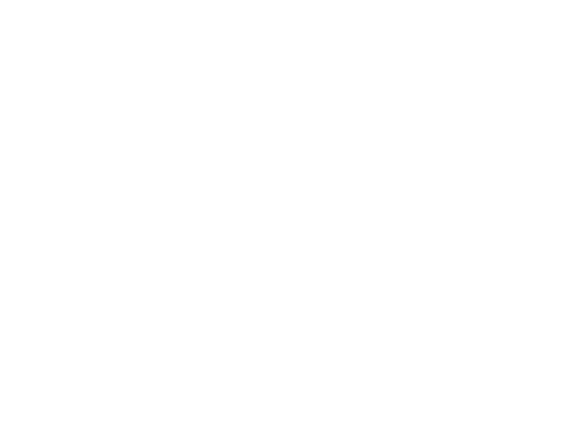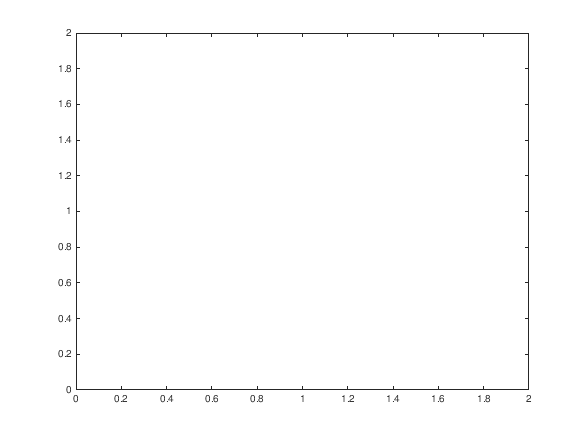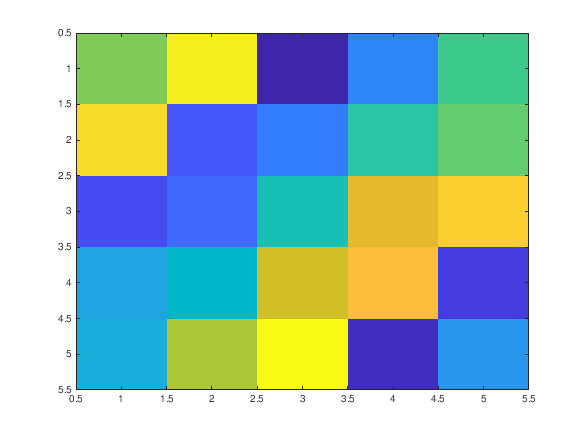Cody

# Problem 43021. How many figures currently exist?

Solution 1150966

Submitted on 31 Mar 2017
This solution is locked. To view this solution, you need to provide a solution of the same size or smaller.

### Test Suite

Test Status Code Input and Output
1   Fail
assert(isequal(numfig(),0))

Undefined function 'minus' for input arguments of type 'matlab.ui.Figure'. Error in numfig (line 3) y=y-1; Error in TestPoint1 (line 1) assert(isequal(numfig(),0))

``
2   Fail
close all; figure; assert(isequal(numfig(),1))

Undefined function 'minus' for input arguments of type 'matlab.ui.Figure'. Error in numfig (line 3) y=y-1; Error in TestPoint2 (line 3) assert(isequal(numfig(),1))

``
``
3   Fail
close all; figure; figure; assert(isequal(numfig(),2))

Undefined function 'minus' for input arguments of type 'matlab.ui.Figure'. Error in numfig (line 3) y=y-1; Error in TestPoint3 (line 4) assert(isequal(numfig(),2))

``
``
``
4   Fail
close all; figure; figure; plot(1,1); figure; imagesc(magic(5)); assert(isequal(numfig(),3))

Undefined function 'minus' for input arguments of type 'matlab.ui.Figure'. Error in numfig (line 3) y=y-1; Error in TestPoint4 (line 5) assert(isequal(numfig(),3))

``
``
``
``
5   Fail
close all; n = randi(20); for ii=1:n figure; end assert(isequal(numfig(),n))

Undefined function 'minus' for input arguments of type 'matlab.ui.Figure'. Error in numfig (line 3) y=y-1; Error in TestPoint5 (line 6) assert(isequal(numfig(),n))

6   Fail
close all; assert(isequal(numfig(),0))

Undefined function 'minus' for input arguments of type 'matlab.ui.Figure'. Error in numfig (line 3) y=y-1; Error in TestPoint6 (line 2) assert(isequal(numfig(),0))

### Community Treasure Hunt

Find the treasures in MATLAB Central and discover how the community can help you!

Start Hunting!